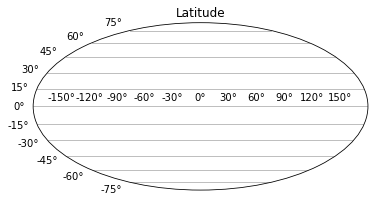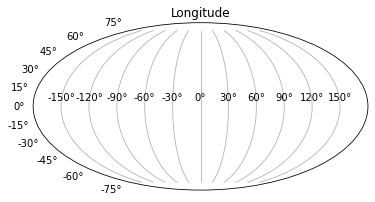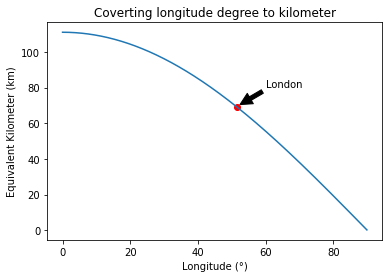import numpy as np
import matplotlib.pyplot as plt


## Latitude#

Latitude is the angular distance of a place north or south of the earth’s equator, or of the equator of a celestial object, usually expressed in degrees, minutes and seconds.

$$1$$ degree latitude is about $$111\,km$$ anywhere on earth.

Lines of latitude are numbered from $$0°$$ at the equator to $$90°N$$ or $$+90$$ at the North Pole. Lines of latitude are numbered from $$0°$$ at the equator to $$90°S$$ or $$-90$$ at the South Pole.

plt.figure()
plt.subplot(111, projection="mollweide")
plt.title("Latitude")
plt.grid(True, axis='y')## Longitude#

The distance of $$1$$ degree longitude varies from $$111\,km$$ at equator to $$0$$ at poles, half way approx. = $$55.8\,km$$ at $$60$$ degrees latitude.

Longitude varies as $$\cos \theta$$, where $$\theta$$ is the latitude (e.g. long at $$80°N = 111\cos(80°) = 19\,km$$)

Lines of longitude begin at the Prime Meridian (Greenwich).

$$60°W$$ is the $$60°$$ line of longitude west of the Prime Meridian. Is also written as $$-60°$$

$$60°E$$ is the $$60°$$ line of longitude east of the Prime Meridian. Is also written as $$+60°$$

Sometimes you will see longitude on a scale $$0°$$ to $$360°$$. The scale is measured clockwise (so $$10°W == 350° == -10°$$)

plt.figure()
plt.subplot(111, projection="mollweide")
plt.title("Longitude")
plt.grid(True, axis='x')# Plot to covert the distance of 1 degree at a certain longitude to kilometer

x = np.arange(0, 90, 0.1)
y = 111*np.cos(x*(np.pi)/180)

plt.plot(x, y)
plt.scatter(51.5, 111*np.cos(51.5*(np.pi)/180),color='r')
plt.annotate('London', xy=(52, 70), xytext=(60, 80),
arrowprops=dict(facecolor='black', shrink=0.05),
)
plt.title("Coverting longitude degree to kilometer")
plt.xlabel('Longitude (°)')
plt.ylabel('Equivalent Kilometer (km)')
plt.show()# The distance of 1 degree at a certain longitude to kilometer convertion
def longitude_km_convertion(longitude):
longitude_km = 111*np.cos(longitude*(np.pi)/180)
return longitude_km

print(f'The distance of 1 degree at 80°N is equal to {round(longitude_km_convertion(80),2)} km.')

The distance of 1 degree at 80°N is equal to 19.27 km.


## Degree Notation#

One degree is equal to $$60$$ minutes and equal to $$3600$$ seconds:

$1° = 60' = 3600''$

One minute is equal to $$1/60$$ degrees:

$1' = (1/60)° = 0.01666667°$

One second is equal to $$1/3600$$ degrees:

$1'' = (1/3600)° = 2.77778e-4° = 0.000277778°$
# Degree notation to decimal degree convertion
def decimal_degree_covertion(degree, minute, second):
decimal_degree = degree + minute/60 + second/3600
return decimal_degree

print(f'53° 30\' 36" is equal to {decimal_degree_covertion(53, 30, 36)}°.')

53° 30' 36" is equal to 53.51°.


### Reference#

2022 notes and practical from Lecture 1 of the module ESE 60028 Tectonics of the Ocean